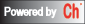## Frequency-Domain Analysis

The frequency response is the system's response to sinusoidal inputs. The output of a linear time-invariant system to a sinusoidal input is a sinusoid of the same frequency but with a different magnitude and phase. The magnitude and phase differences between the input and output sinusoids are functions of frequency.

The most common ways to represent frequency reponse of a system are Bode plot and Nyquist plot. A Bode plot shows the relationship between the magnitude and phase values of the open-loop transfer function G(j*w) and input frequency(when the input frequency w goes from zero to infinity). A Nyquist plot is a representation of open-loop transfer function G(j*w) in s plane. The Nyquist plot allows us to predict the stability performance of a closed-loop system by observing its open-loop behavior.

The functions below can be used to plot the Bode plot, Nyquist plot, and Nichols chart, to claculate the gain and phase margin of a system or to calculate the value of open-loop transfer function over a given frequency vector.

Function
`    `
Description
Bode diagram Plot the Bode frequency response of a system.
Gain and phase margin Plot the gain and phase margins of a system.
Nichols chart Plot the Nichols frequency response of a system.
Nyquist diagram Plot the Nyquist frequency response of a system.
Frequency response Calculate the system frequency response.